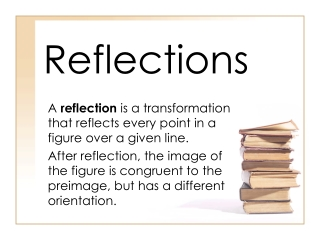DownloadDownload PresentationReflections

# Reflections

Télécharger la présentation## Reflections

- - - - - - - - - - - - - - - - - - - - - - - - - - - E N D - - - - - - - - - - - - - - - - - - - - - - - - - - -
##### Presentation Transcript

1. Reflections A reflection is a transformation that reflects every point in a figure over a given line. After reflection, the image of the figure is congruent to the preimage, but has a different orientation.

2. Property of reflection • A reflection is an isometry, meaning the preimage and its reflected image have the same size and shape.

3. To reflect a point across a horizontal or vertical line, imagine that the line is a mirror, and visualize the reflected location of the point.

4. Reflecting across an axis • Reflect tri ABC across the y-axis • Imagine each point reflected across a mirror sitting on the y-axis. When a point is reflected across the y-axis , its y-coordinate does not change

5. Reflecting across a horizontal line • Reflect tri ABC across the x-axis • Notice that when a point is reflected across a horizontal line, its x-coordinate does not change

6. Reflecting across a line • To find the reflection of a point across any line in the coordinate plane, draw a perpendicular line from the point to the line of reflection. The point's reflection will be equidistant from the line of reflection on both sides

7. practice • Rectangle ABCD has vertices at A(1,1), B(5.5,1), C(5.5,3.5), D(1,3.5) • Reflect ABCD across the y-axis • Reflect ABCD across the line y = 2 • Reflect ABCD across the line y =x